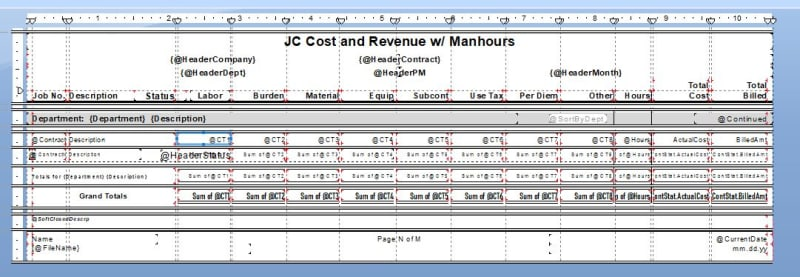×
INTELLIGENT WORK FORUMS
FOR COMPUTER PROFESSIONALS

Are you a
Computer / IT professional?
Join Tek-Tips Forums!
• Talk With Other Members
• Be Notified Of Responses
• Keyword Search
Favorite Forums
• Automated Signatures
• Best Of All, It's Free!

*Tek-Tips's functionality depends on members receiving e-mail. By joining you are opting in to receive e-mail.

#### Posting Guidelines

Promoting, selling, recruiting, coursework and thesis posting is forbidden.

# why is my result getting multiplied by 17??

## why is my result getting multiplied by 17??

(OP)
thread767-1267707: why is my result getting multiplied by 10????

I have a report i am writing, pulling info from a SQL table.

The Sum of each cost type is multiplying by 17. For example the total should result in $1,260,415 and I'm getting$21,427,055. 17 is the number of subcontracts for this particular job.

Below is the formula for all of the cost types.

Sum ({@CT1}, {brptJCCostRev;1.Contract})=0 and
Sum ({@CT2}, {brptJCCostRev;1.Contract})=0 and
Sum ({@CT3}, {brptJCCostRev;1.Contract})=0 and
Sum ({@CT4}, {brptJCCostRev;1.Contract})=0 and
Sum ({@CT5}, {brptJCCostRev;1.Contract})=0 and
Sum ({@CT6}, {brptJCCostRev;1.Contract})=0 and
Sum ({@CT7}, {brptJCCostRev;1.Contract})=0 and
Sum ({@CT8}, {brptJCCostRev;1.Contract})=0 and
Sum ({@CT9}, {brptJCCostRev;1.Contract})=0 and
Sum ({brptJCCostRev;1.ActualCost}, {brptJCCostRev;1.Contract})=0 and
Sum ({brptJCCostRev;1.BilledAmt}, {brptJCCostRev;1.Contract})=0 and
{?ShowZeros}='N'

In another thread, the user figured out that there was no filter in place to limit the sub contracts it was pulling amounts from, so it was pulling the amounts for everything - 10 times (b/c there were 10 sub contracts). How do I set this filter to only take the one total of the cost type and not multiply it by the number of subcontracts?

### RE: why is my result getting multiplied by 17??

It's hard to be specific with a solution based on the information provided, but the inclusion of the subcontracts has resulted in the detail section to be repeated for each of them which means the sum is 17 times higher.

You could use either a Running Total or a Variable so as to accumulate the total only once for each contract.

If you require more specific instructions you will need to provide more details on the report structure (grouping, location of the formula provided, details of 9 @CT× formulas etc.)

Hope this helps.

Cheers, Pete

### RE: why is my result getting multiplied by 17??

(OP)
See below for the Section Expert. The Formulas I posted above are coming from "Group Footer #3: brptJCCostRev;1.Contract - A" Where would I got to put in a Running Total of a Variable?

Details
Group Footer #3
Group Footer #2
Group Footer #1
Page Footer
Page Footer a
Page Footer b
Report Footer

### RE: why is my result getting multiplied by 17??

Sorry, my earlier post should have said Running Total OR a Variable.

I am still a bit confused by the "= 0" against each of the formulas above and the reference to "{?ShowZeros}='N'".

Is what you have shown above, the contents of another formula, a Group Selection formula or simply a typo?

Assuming the 11 formulas above are all sitting in GF3, Grp 3 is {brptJCCostRev;1.Contract}), there are 17 rows of data (details section), all showing the same values for {@CT1}, {@CT2} ... etc, you could simply drag each of those {@CTx} formulas to GF3.

Does that help?

If you then need to add each of those amounts for totals in Grp2, Grp1 etc, that will require some additional work which I am happy to help with but I need to be sure I understand the situation before investing additional time to assist.

If you are happy to post a copy of the report with saved data, it might be easier than trying to explain it, but I understand you may not be prepared to do that.

Cheers,
Pete.

### RE: why is my result getting multiplied by 17??

(OP)So in the Detail Section their are the cost types: @CT1, @CT2, @CT3, etc.
Each of these have the following formula:

if {brvJCContStat.CostType} = 1 then {brvJCContStat.ActualCost} else 0

So how do I edit this formula so that each Cost Type is not multiplying the correct amount by 17 (the number of subcontracts for this job)

### RE: why is my result getting multiplied by 17??

Actually, what I asked for was to post the report with saved data, not a screenshot.

A screenshot showing the section names might help.

### RE: why is my result getting multiplied by 17??

No sorry, the link doesn't seem to have worked.

#### Red Flag This Post

Please let us know here why this post is inappropriate. Reasons such as off-topic, duplicates, flames, illegal, vulgar, or students posting their homework.

#### Red Flag Submitted

Thank you for helping keep Tek-Tips Forums free from inappropriate posts.
The Tek-Tips staff will check this out and take appropriate action.

Close Box

# Join Tek-Tips® Today!

Join your peers on the Internet's largest technical computer professional community.
It's easy to join and it's free.

Here's Why Members Love Tek-Tips Forums:

•Talk To Other Members
• Notification Of Responses To Questions
• Favorite Forums One Click Access
• Keyword Search Of All Posts, And More...

Register now while it's still free!